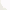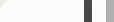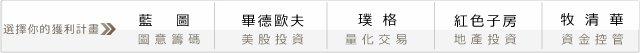## 程式獵人知識庫－淺談資料對程式的影響#### 回測時間，SHOW TIME!

(1)當短期（4根K棒）移動平均線由下往上突破長期（18根K棒）移動平均線時，

(2) 當短期（4根K棒）移動平均線由下往上突破中期（9根K棒）移動平均線時，

(2)到期當天出場，等到下次訊號出現時在進場，此方式可以避免到期換月問題。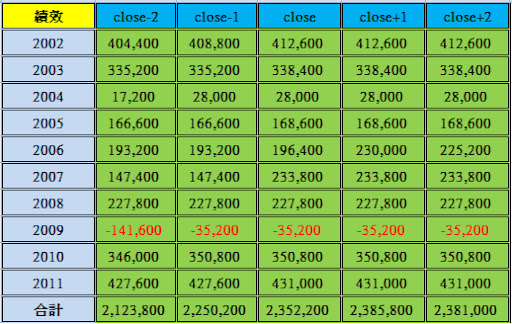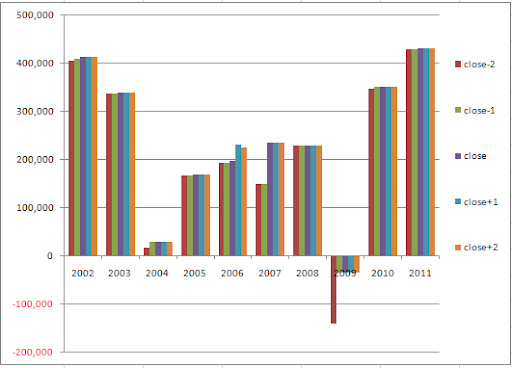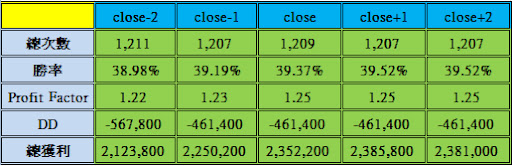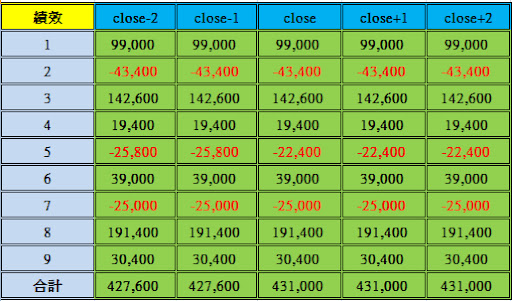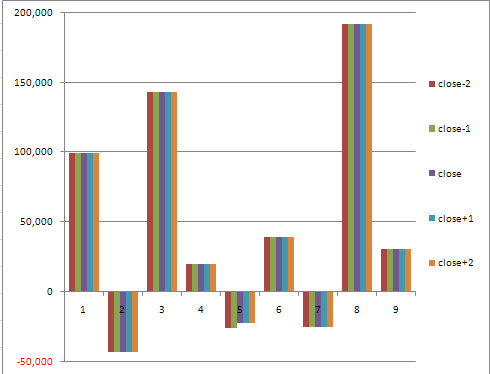if date <> date then begin
TodayHigh = 0;
TodayLow = 99999;
SellFlag = False; 獵人在之前的文章這邊不小心多打了這兩個條件，

}
TodayHigh=opend(0)+avgtruerange(6);
TodayLow =opend(0)-avgtruerange(6);
end;

if barnumber=1 then aa=0;

newclose=close;

if barnumber=60*(1+aa) then begin
aa=aa+1;
newclose=close-2;
end;

if AverageFC(newclose,4) cross over AverageFC(newclose,9) then begin
s=1;
u=0;
end;

if AverageFC(newclose,4) cross under AverageFC(newclose,9) then begin
s=0;
u=1;
end;

if s=1 and AverageFC(newclose,4) cross over AverageFC(newclose,18) then begin
SellFlag=false;
end;

if u=1 and AverageFC(newclose,4) cross under AverageFC(newclose,18) then begin
SellFlag=true;
end;

if time<1330 and buyflag=true and high > Todayhigh then buy next bar at highest(high,3)+1 stop;

if time<1330 and sellflag=true and low < Todaylow then sell next bar at lowest(low,3)-1 stop;

if marketposition>0 then exitlong next bar at entryprice-80 stop;

if marketposition<0 then exitshort next bar at entryprice+80 stop;

Condition1=(dayofweek(date)=3 and dayofmonth(date) > 14 and 22 > dayofmonth(date)) or date=1040127 or date=1070226 or date=1100617 or date=1100222;
if condition1=true then begin
if time>1314 then begin
exitlong on close;
exitshort on close;
end;
end;

#### 0 意見: